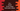# C++ program to check if two arrays hold the same values## C++ program to check if two arrays hold the same values:

In this post, we will learn how to check if two arrays are equal or not in C++. The program will take the numbers of each array as inputs from the user and print one message if both arrays are equal or not.

### Algorithm to check if two arrays hold the same values:

The easiest way to solve this is by sorting both arrays and iterate them parallely. This approach is easy to implement and we can compare both arrays.

### C++ program to check if two arrays hold same values:

Below is the complete C++ program:

``````#include <iostream>
#include <algorithm>

using namespace std;

bool compareArrays(int firstArray[], int secondArray[], int arraySize)
{
bool found;

sort(firstArray, firstArray + arraySize);
sort(secondArray, secondArray + arraySize);

for (int i = 0; i < arraySize; i++)
{
if (firstArray[i] != secondArray[i])
{
return false;
}
}
return true;
}

int main()
{
int arraySize;

cout << "Enter the size of the arrays :" << endl;
cin >> arraySize;

int firstArray[arraySize], secondArray[arraySize];

cout << "Enter the values for the first array :" << endl;
for (int i = 0; i < arraySize; i++)
{
cout << "Enter the value for index " << i + 1 << " : " << endl;
cin >> firstArray[i];
}

cout << "Enter the values for the second array :" << endl;
for (int i = 0; i < arraySize; i++)
{
cout << "Enter the value for index " << i + 1 << " : " << endl;
cin >> secondArray[i];
}

if (compareArrays(firstArray, secondArray, arraySize))
{
cout << "Both arrays are equal" << endl;
}
else
{
cout << "Both arrays are not equal" << endl;
}
}``````

#### Explanation:

In this program,

• arraySize is used to hold the size of the array. Both arrays are of equal size.
• Using two for loops, we are reading the contents for the arrays. firstArray is used to hold the contents of the first array and secondArray for the second array.
• compareArrays method is used to compare two arrays. It takes two arrays and the array size and returns one boolean. true if both arrays are equal and false if not.
• compareArrays first sort both arrays and then using a for loop, it compares the contents of both arrays and returns false if any two values are not equal. If all are equal, then it returns true.
• Based on the result of compareArrays, we are printing one message that the arrays are equal or not.

### Sample output:

It will give output as like below:

``````Enter the size of the arrays :
4
Enter the values for the first array :
Enter the value for index 1 :
1
Enter the value for index 2 :
2
Enter the value for index 3 :
3
Enter the value for index 4 :
4
Enter the values for the second array :
Enter the value for index 1 :
4
Enter the value for index 2 :
3
Enter the value for index 3 :
2
Enter the value for index 4 :
1
Both arrays are equal``````